# NCERT Solutions for Class 8 Maths Chapter 2 Linear Equations in One Variable

NCERT Solutions for Class 8 Maths Chapter 2 Linear Equations in One Variable

### NCERT Solutions for Class 8 Maths Chapter 2 Linear Equations in One Variable Exercise 2.1

Ex 2.1 Class 8 Maths Question 1.
Solve the equation: x – 2 = 7.
Solution:
Given: x – 2 = 7
⇒ x – 2 + 2 = 7 + 2 (adding 2 on both sides)
⇒ x = 9 (Required solution)

Ex 2.1 Class 8 Maths Question 2.
Solve the equation: y + 3 = 10.
Given: y + 3 = 10
⇒ y + 3 – 3 = 10 – 3 (subtracting 3 from each side)
⇒ y = 7 (Required solution)

Ex 2.1 Class 8 Maths Question 3.
Solve the equation: 6 = z + 2
Solution:
We have 6 = z + 2
⇒ 6 – 2 = z + 2 – 2 (subtracting 2 from each side)
⇒ 4 = z
Thus, z = 4 is the required solution.

Ex 2.1 Class 8 Maths Question 4.Solution: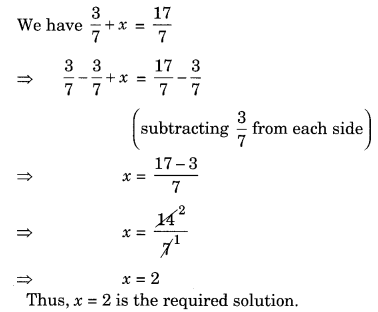Ex 2.1 Class 8 Maths Question 5.
Solve the equation 6x = 12.
Solution:
We have 6x = 12
⇒ 6x ÷ 6 = 12 ÷ 6 (dividing each side by 6)
⇒ x = 2
Thus, x = 2 is the required solution.

Ex 2.1 Class 8 Maths Question 6.Thus, t = 50 is the required solution.

Ex 2.1 Class 8 Maths Question 7.Thus, x = 27 is the required solution.

Ex 2.1 Class 8 Maths Question 8.Thus, y = 2.40 is the required solution.

Ex 2.1 Class 8 Maths Question 9.
Solve the equation 7x – 9 = 16.
Solution:Ex 2.1 Class 8 Maths Question 10.Ex 2.1 Class 8 Maths Question 11.
Solve the equation 17 + 6p = 9.
Solution:Ex 2.1 Class 8 Maths Question 12.Solution: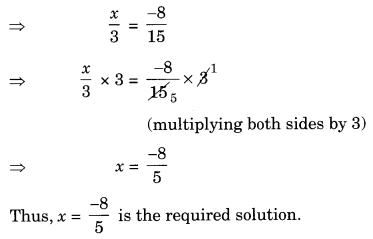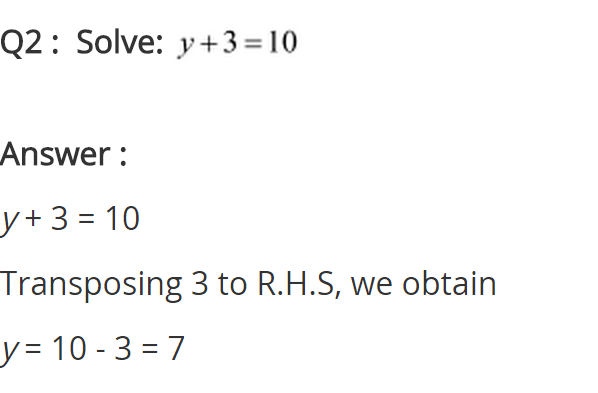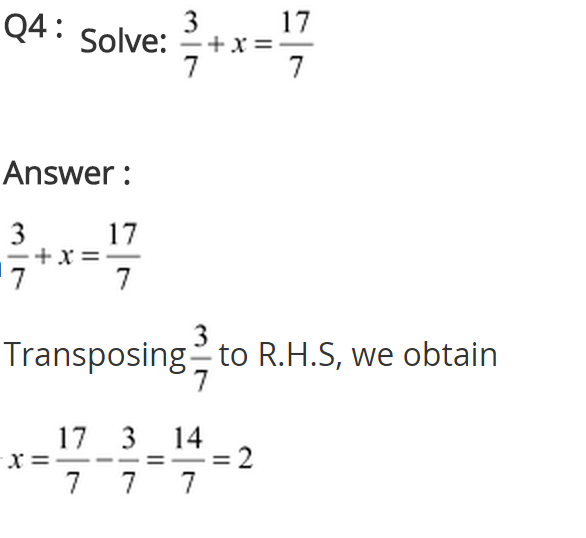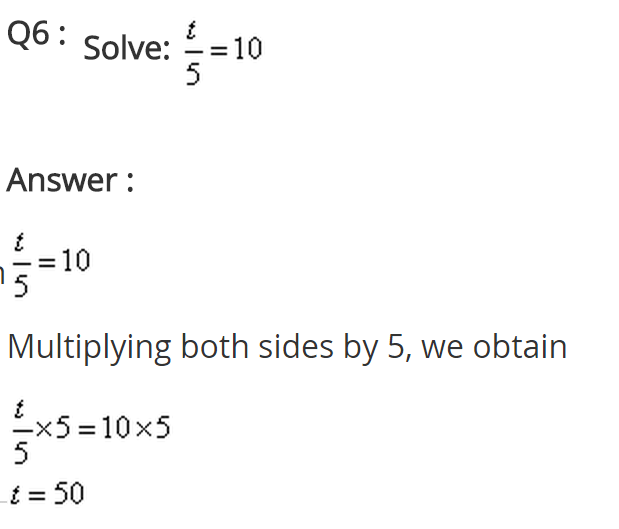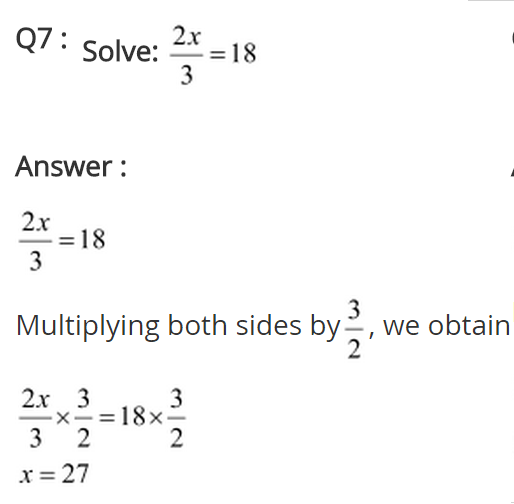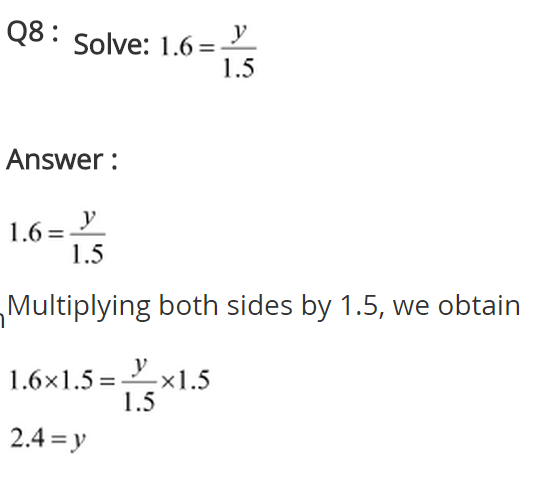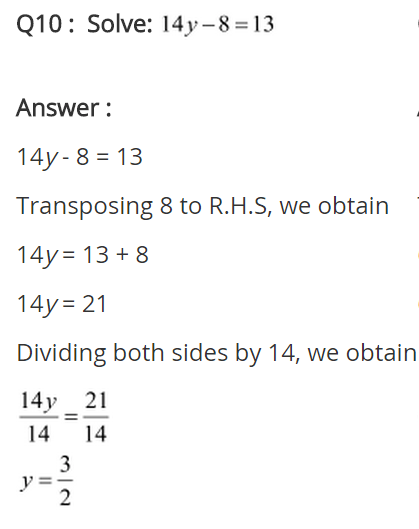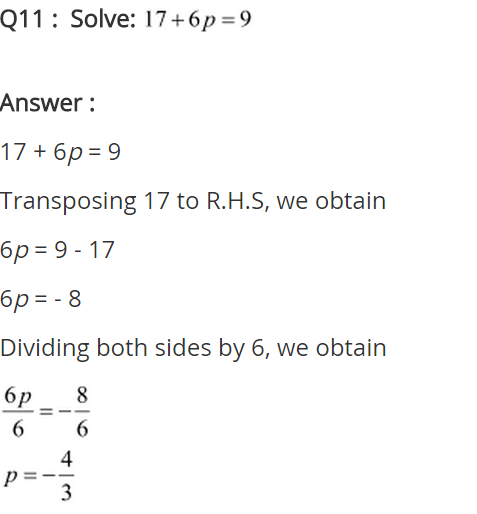## SabDekho

The Complete Educational Website